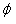Courses

# Test: Motional Emf

## 10 Questions MCQ Test Physics Class 12 | Test: Motional Emf

Description
This mock test of Test: Motional Emf for JEE helps you for every JEE entrance exam. This contains 10 Multiple Choice Questions for JEE Test: Motional Emf (mcq) to study with solutions a complete question bank. The solved questions answers in this Test: Motional Emf quiz give you a good mix of easy questions and tough questions. JEE students definitely take this Test: Motional Emf exercise for a better result in the exam. You can find other Test: Motional Emf extra questions, long questions & short questions for JEE on EduRev as well by searching above.
QUESTION: 1

### The magnetic flux passing normally through a coil is ø = 20 cos πt + t2 + 50t + 25m Wb What will be the induced emf at t = 1s?​

Solution:

θ=20cos5πt+t2+50t+25 mWb
We know that,
Emf=dθ/dt=-20x5πsin5πt+2t+50+0
At,t=1
Emf=-100πsing5π+2+50
=0+2+50
=52V

QUESTION: 2

### A copper rod moves parallel to the horizontal direction. The induced emf developed across its ends due earth’s magnetic field will be maximum at the

Solution:

At the poles of the copper rod, the earth’s magnetic field will be more. This is explained with the help of Lorentz force where he explains that the magnetic field is generated due to the movement of charges.

Earth’s magnetic field is defined as the magnetic dipole which is similar to bar magnet and is tilted 11° from the earth’s axis of rotation.

QUESTION: 3

### The magnetic flux through a surface varies with time as follows:= 12t2 + 7t + 3 here,is in milliweber and t is in seconds. What will be the induced emf at t = 5s?​

Solution:

Given :
ϕ=12t2+7t+C
where, C is a constant, t is in sec, ϕ in milli-weber
The induced e.m.f. in the loop at t=5, on differentiating the equation with respect to 't'.
dtdϕ​=dtd​(12t2+7t+C)
=24t+7
at            t=5
dtdϕ​=24×(5)+7
=120+7
=127 mV

QUESTION: 4

In Faraday’s experiment if the magnet is moved towards the coil, it results in ____________ in magnetic field B at any point on the wire loop. The _________ shows deflection. Thus emf is induced by changing B.

Solution:
QUESTION: 5

What is the value of induced e.m.f if a wire is moved between the gap of two poles of a magnetin in 1 s and magnetic flux between the poles is 6 x 10-3 Wb?​

Solution:
QUESTION: 6

Two similar circular co-axial loops carry equal current in the same direction. If the loops be brought nearer, the currents in them will

Solution:

When the two loops are brought together, the flux linking the two loops increases. By Lenz's law a current will be induced such that it opposes this change of flux . Therefore to oppose the increase in flux, the current in the two loops will decrease, so the flux decreases.

QUESTION: 7

When a wire loop is rotated in a magnetic field, the direction of induced emf changes once in each

Solution:

Flux of the magnetic field through the loop
ϕ=B.Acosωt
Emf= −dϕ/dt​=B.Aωsinωt
ωt=0 to π,  sinωt=+ve
ωt=π to2 π,  sinωt=−ve
Hence, the direction of induced e.m.f. changes once in 1/2​ revolution.

QUESTION: 8

The induced e.m.f in a coil is given by ε = 100sin 100π volts. Calculate the number of rotations the coil makes per second.​

Solution:

ε=100sinπV
ε=ε0sinωt
ω=100π
2πn=100π
n=50rps

QUESTION: 9

The motional emf produced across a wire moving through a magnetic field does not depend on its:

Solution:

Motional emf= Bℓv
It depends on magnetic field intensity, length and velocity. The diameter doesn't affect any of the factors.

QUESTION: 10

If we keep two similar loops made up of copper and constantan in a magnetic field for same amount of time, in which loop the induced e.m.f will be greater.

Solution:

As the copper has high electrical conductivity. Hence, higher e.m.f would be induced in the copper loop.